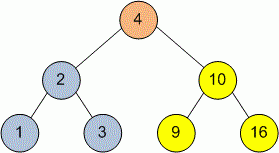Competitions

# Tree InOrder Traversal

Implement an InOrder traversal of a tree. In this traversal left subtree is visited first, then root node, and finally right subtree.

Definition of a tree:

// Java
class TreeNode
{
public:
int val;
TreeNode left;
TreeNode right;
TreeNode(int x) {
val = x;
left = NULL;
right = NULL;
};

// C++
class TreeNode
{
public:
int val;
TreeNode *left;
TreeNode *right;
TreeNode(int x) : val(x), left(NULL), right(NULL) {}
};


Implement function InOrder that prints in one line the vertices of the tree in order of InOrder traversal. Separate the vertices with one space.

// Java
void InOrder(TreeNode tree)

// C++
void InOrder(TreeNode *tree)


#### ExampleDuring the InOrder traversal vertices will be printed in the next order: 1 2 3 4 9 10 16.

Time limit 1 second
Memory limit 128 MiB
Author Mykhailo Medvediev